8.2

## Lecture 9: Proper Tail Calls: Using the stack better

### 1A motivating example

A compiler’s job is to faithfully translate the semantics of one language into another; this much is obvious. However, not all translations are equal: some can be drastically more efficient than others, to the point where they change which programs we can actually, effectively run. This is not a claim about optimization, though. Optimizations typically improve the performance by some constant factor, meaning the amount of a particular resource (time, memory, IO, etc.) is lowered by some fraction. Our concern here is about compiling a ubiquitous feature of our language—function calls—in such a way that it provides an asymptotic improvement in our program.

Here is a function from the interp.rs for looking up values in the environment implemented as a linked list:

enum List<T> {
Empty,
Cons(T, Rc<List<T>>),
}

fn get(stk: &List<(&str, i64)>, x: &str) -> Option<i64> {
match stk {
List::Empty => None,
List::Cons((y, n), stk) => {
if x == *y {
Some(*n)
} else {
get(stk, x)
}
}
}
}

Here Rc<T> is a reference-counted pointer, but for this example just think of it as Box<T>.

What are the practical limits of this function? Conceptually, it should work for any list we’ve constructed. But in practice, this code might crash on lengthy lists, simply because the recursion progressed too deeply: we encounter a stack overflow, because we can’t allocate a stack frame for the next recursive call. This is dissatisfying: our language semantics don’t include any arbitrary limits on the depth of recursion. And clearly, whatever machine we ran this program on was capable of building a long list; it seems capricious to then be unable to process it!

Looking more carefully at the program, though, this failure is even more disappointing. When the if condition is false, we fall through to the else-branch and start evaluating the recursive call to get. But notice that when that call returns...the function simply passes the return value back as its answer, with no further processing needed! It makes sense that we’d need a stack frame to handle the recursive call, but the current stack frame is basically no longer needed. Perhaps we could consolidate the two somehow, and not need any extra space?

### 2A simpler example

Let’s consider a program in Diamondback that has a similar recursive structure. Instead of working through a list data structure (which we do not yet have), let’s compute the factorial function:

def fact_v1(n):
if n <= 1: 1
else: n * fact_v1(n - 1)
end

At first glance, this doesn’t match the structure of get since here we have additional work to do after the recursive call to fact(n - 1). But we’ve learned ways to transform this program into a similar one, such that the answer to the recursive call simply is the overall answer: we use an accumulator parameter, and rewrite the code as follows:

def fact-tail(n, acc):
if n <= 1: acc
else: fact-tail(n - 1, n * acc)
end

def fact_v2(n):
fact-tail(n, 1)
end

Compare and contrast the evaluation order of these two functions, using the substitution model of evaluation that we began the course with:

fact_v1(4) ==> if 4 <= 1: 1 else 4 * fact_v1(3)
==> 4 * fact_v1(3)
==> 4 * (if 3 <= 1: 1 else 3 * fact_v1(2))
==> 4 * (3 * fact_v2(2))
==> 4 * (3 * (if 2 <= 1: 1 else 2 * fact_v1(1)))
==> 4 * (3 * (2 * fact_v1(1)))
==> 4 * (3 * (2 * (if 1 <= 1: 1 else 1 * fact_v1(0))))
==> 4 * (3 * (2 * (1)))
==> 4 * (3 * 2)
==> 4 * 6
==> 24

fact_v2(4) ==> fact_tail(4, 1)
==> if 4 <= 1: 1 else fact-tail(4 - 1, 4 * 1)
==> fact_tail(3, 4)
==> if 3 <= 1: 4 else fact-tail(3 - 1, 3 * 4)
==> fact_tail(2, 12)
==> if 2 <= 1: 12 else fact-tail(2 - 1, 2 * 12)
==> fact_tail(1, 24)
==> if 1 <= 1: 24 else fact-tail(1 - 1, 1 * 24)
==> 24

The initial version keeps a bunch of multiplications pending, until the innermost function call returns. It is reasonable to think that in our compiled code, each of those will correspond to one stack frames, and we clearly still need to keep track of the intermediate values of n in order to compute the final answer.

The second version of the function, though, never has more than one call to fact-tail pending. Nothing in this evaluation sequence “looks like” it needs a deep call stack. Can we achieve this?

### 3Defining tail position

What distinguishes the recursive calls to fact_v1 from the calls to fact_tail (or, for that matter, get)? Intuitively, we described them as “the last thing to be done”, before returning from the function. We say that such expressions are in tail position, and we can define such positions explicitly, looking at each expression form in our language:

1. The expression of our program is in tail position.

2. The body of a function is in tail position.

3. If a let-binding is in tail position, then (a) its body is in tail position, but (b) the bindings themselves are not.

4. If a conditional is in tail position, then (a) its branches are in tail position, but (b) the condition itself is not.

5. The operands to an operator are never in tail position.

Visually, green expressions are always in tail position, yellow expressions are potentially in tail position, and red expressions are never in tail position:

pub struct Prog<E, Ann> {
pub funs: Vec<FunDecl<E, Ann>>,
pub ~hl:3:s~main: E~hl:3:e~
pub ann: Ann,
}
pub struct FunDecl<E, Ann> {
pub name: String,
pub parameters: Vec<String>,
pub ~hl:3:s~body: E~hl:3:e~,
pub ann: Ann,
}
pub enum SeqExp<Ann> {
Imm(~hl:2:s~ImmExp~hl:2:e~, Ann),
Prim1(Prim1, ~hl:2:s~ImmExp~hl:2:e~, Ann),
Prim2(Prim2, ~hl:2:s~ImmExp~hl:2:e~, ~hl:2:s~ImmExp~hl:2:e~, Ann),
Let {
var: String,
~hl:2:s~bound_exp: Box<SeqExp<Ann>>~hl:2:e~,
~hl:1:s~body: Box<SeqExp<Ann>>~hl:1:e~,
ann: Ann,
},
If {
~hl:2:s~cond: ImmExp~hl:2:e~,
~hl:1:s~thn: Box<SeqExp<Ann>>~hl:1:e~,
~hl:1:s~els: Box<SeqExp<Ann>>~hl:1:e~,
ann: Ann,
},
~hl:1:s~Call~hl:1:e~(String, ~hl:2:s~Vec<ImmExp>~hl:2:e~, Ann),
}

We can codify this, if we so choose, as a kind of tagging operation:

fn mark_tails<Ann>(e: &SeqExp<Ann>, is_tail: bool) -> SeqExp<bool> {
match e {
SeqExp::Imm(i, _) => SeqExp::Imm(i.clone(), is_tail),
SeqExp::Prim1(op, i, _) => SeqExp::Prim1(*op, i.clone(), is_tail),
...
SeqExp::Let {
var,
bound_exp,
body,
..
} => SeqExp::Let {
var: var.clone(),
bound_exp: Box::new(mark_tails(bound_exp, false)),
body: Box::new(mark_tails(&body, is_tail)),
ann: is_tail,
},
SeqExp::If { cond, thn, els, .. } => SeqExp::If {
cond: cond.clone(),
thn: Box::new(mark_tails(thn, is_tail)),
els: Box::new(mark_tails(els, is_tail)),
ann: is_tail,
},
}
}

In practice we probably don’t need to, and instead can just carry along a boolean flag through our compile_with_env function that keeps track of our tail-position status:

fn compile_with_env<'exp>(
e: &'exp SeqExp<u32>,
.., // other arguments
is_tail: bool) // true when the expression is in tail position
-> Vec<Instr> {
match e {
...
SeqExp::Let { var, bound_exp, body, ann } => {
...
let bound_exp_is = compile_with_env(bound_exp, ..., false);
...
let body_is = compile_with_env(body, ..., is_tail)
}
SeqExp::Call(fun, args, ann) => {
if is_tail {
// generate a tail call
} else {
// generate a non-tail call
}
}
...
}
}

### 4Examining the stack

Let’s consider what the stack looks like while evaluating fact_v1. In this diagram, colors indicate which stack frame uses a particular value on the stack, while the brackets indicate which stack frame created a particular value on the stack. In this example we are using the stack-only calling convention, where all function arguments are pushed onto the stack.

 In top-level In fact_v1(4) In fact_v1(3) In fact_v1(2) In fact_v1(1) At fact_v1(4) At fact_v1(3) At fact_v1(2) At fact_v1(1) About to return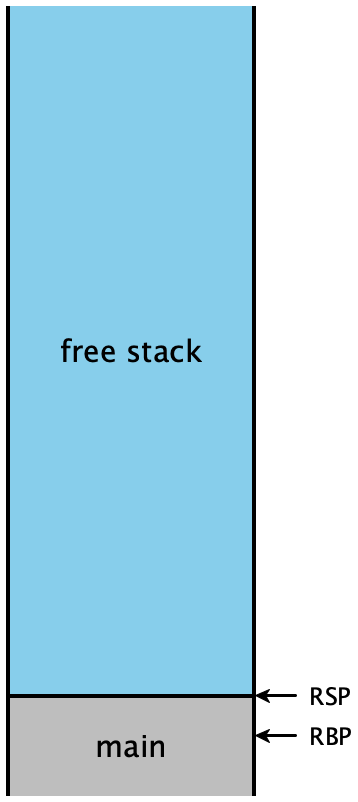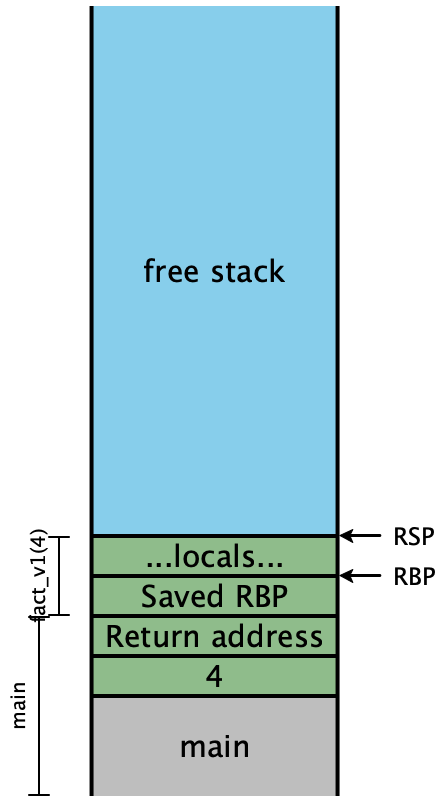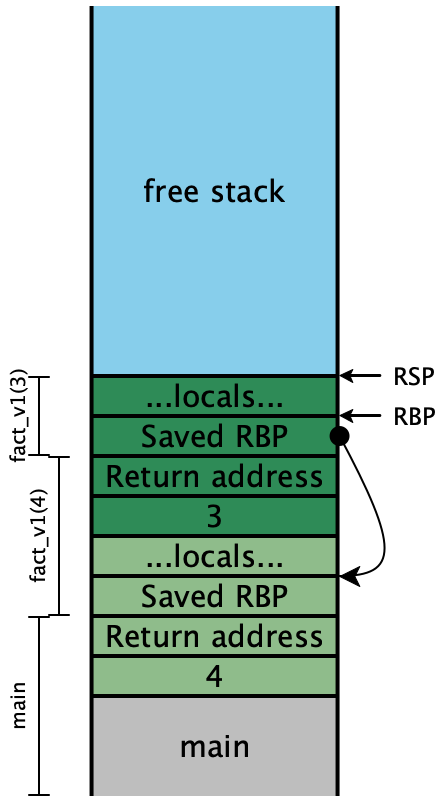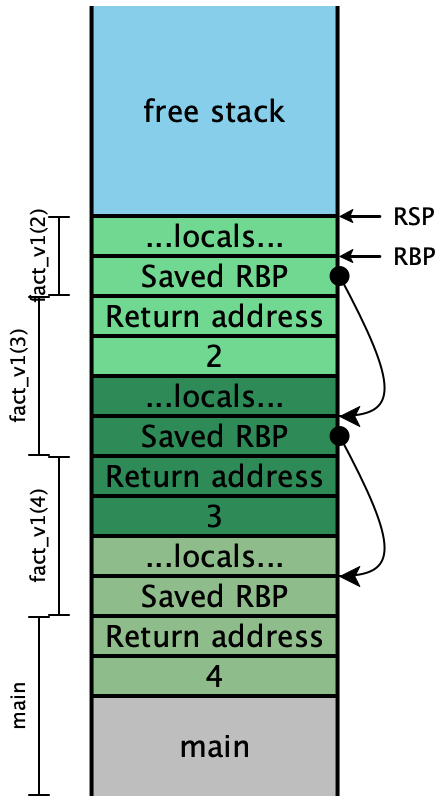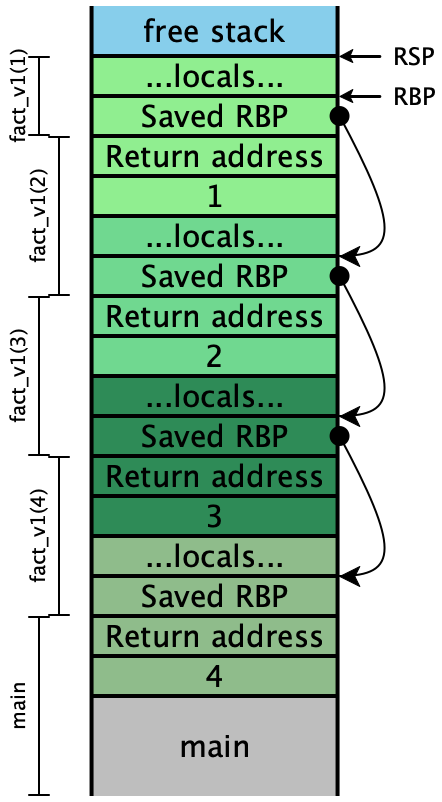Now let’s examine the stacks for fact_v2, assuming we compile our code exactly as we’ve always been. We’ll include the local variables, this time:

 In top-level In fact_tail(4, 1) In fact_tail(3, 4) In fact_tail(2, 12) In fact_tail(1, 24) At fact_tail(4, 1) At fact_tail(3, 4) At fact_tail(2, 12) At fact_tail(1, 24) About to return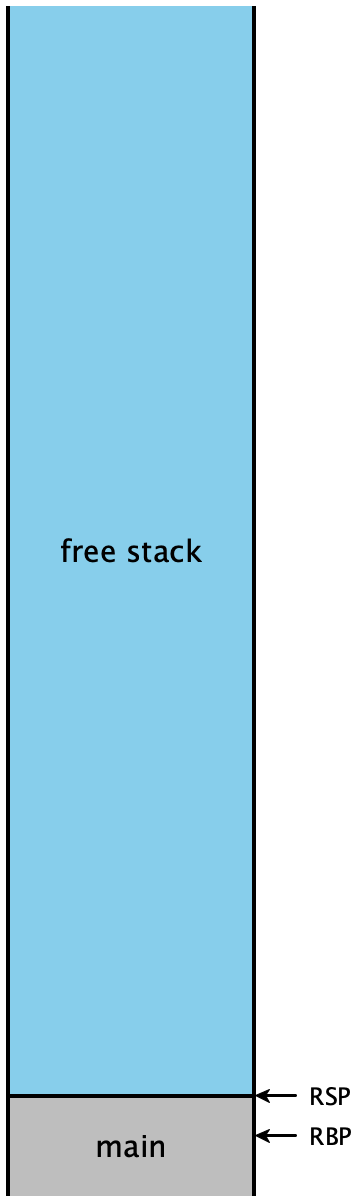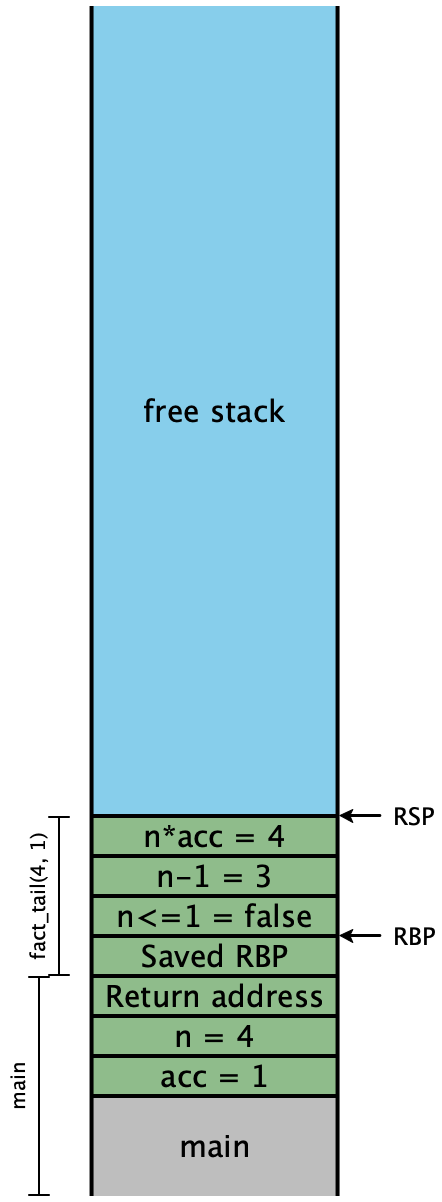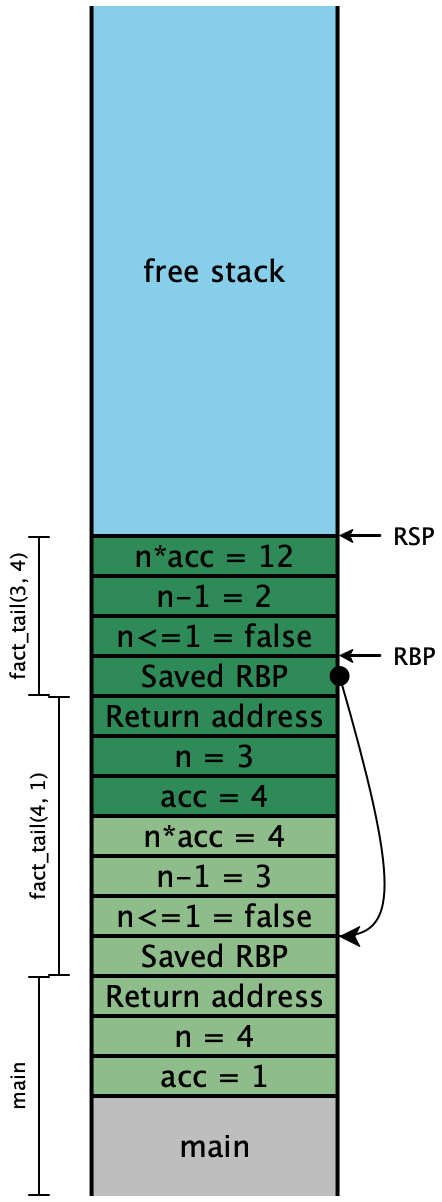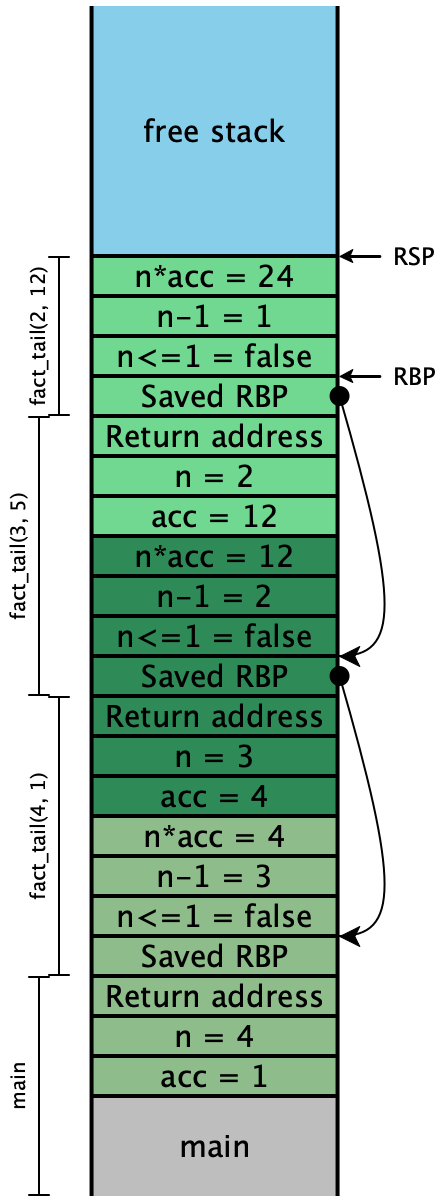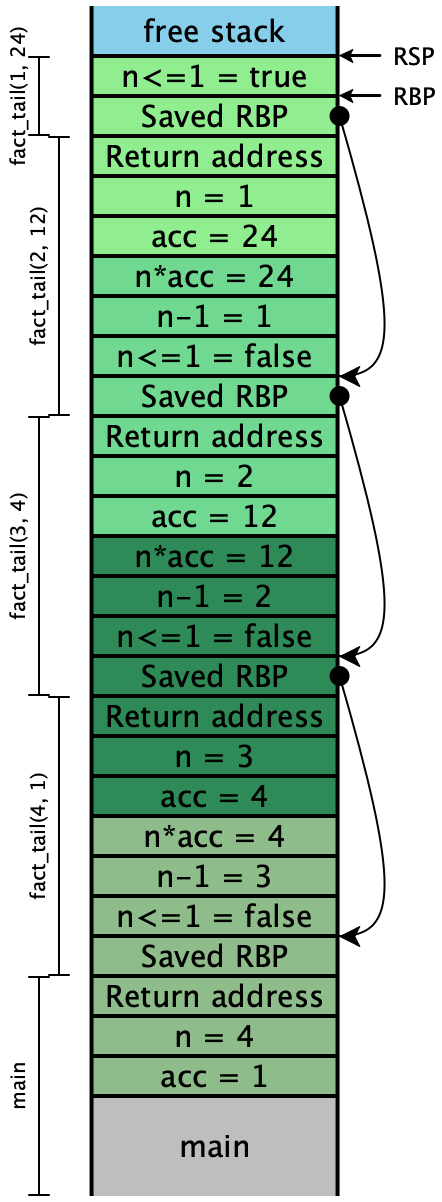Because the recursive calls here are all in tail-position, the next four instructions are all going to be ret instructions, which means the entirety of this stack can effectively be eliminated in one step. In other words, once the olive stack frame makes the call to the dark green frame, we never need to access an olive stack slot again. Looking carefully at the stack, we see that the next values for n and acc are precisely the local values computed in the previous stack frame, and moreover, each stack frame has exactly the same shape. If instead of creating a new stack frame, we simply reused the existing one, then we wouldn’t need more than constant stack depth to provide arbitrary call depth!

### 5Strategy

Rather than pushing the next set of arguments onto the stack, simply move them into the existing stack slots at RBP + 16, RBP + 24, etc. Once we’ve done that, we need to re-enter our existing function, but we can’t use call to do it.

Do Now!

Why not?

The meaning of call is to push a return address onto the stack and jump to the destination address. But we already have the necessary return address sitting on the stack! We also have a saved RBP on the stack too, which means that the function prologue we normally execute isn’t really needed here. So instead, we’ll simply jump directly to the next instruction in our code. The compiled assembly for fact_tail would then look roughly like this (ignoring all tag checks, and simplifying the condition slightly):

fact_tail:
fact_tail_prologue:
push RBP
mov RBP, RSP
sub RSP, 16           ; reserve stack slots
fact_tail_body:
mov RAX, [RBP + 16]   ; load n
cmp RAX, 2            ; compare to representation of 1
jg keep_going
mov RSP, RBP          ; and return directly
pop RBP               ; to the original
ret                   ; caller
keep_going:
mov RAX, [RBP + 16]   ; \
sub RAX, 2            ; | compute n - 1
mov [RBP - 8], RAX    ; /
mov RAX, [RBP + 16]   ; \
sar RAX, 1            ; |
imul RAX, [RBP + 24]  ; | compute n * acc
mov [RBP - 16], RAX   ; /
mov RAX, [RBP - 8]    ; \
mov [RBP + 16], RAX   ; / OVERWRITE argument n
mov RAX, [RBP - 16]   ; \
mov [RBP + 24], RAX   ; / OVERWRITE argument acc
jmp fact_tail_body    ; AND RESTART fact_tail

This code is almost legible enough that we could turn it into Rust code pretty easily:

fn fact_tail(mut n: i64, mut acc: i64) -> i64 {
while true {
if n <= 1 {
return acc;
} else {
let tmp1 = n - 1;
let tmp2 = n * acc;
n = tmp1;
acc = tmp2;
}
}
}

We’ve turned our (tail-)recursive function into a while-loop, and eliminated all the function calls!

### 6Implementation pitfalls

#### 6.1Reusing arguments

Consider the following code:

def max(x, y):
if y >= x: y
else: max(y, x)

This is clearly tail-recursive, so we can apply the same technique above. Since we have no intermediate expressions (again, simplifying the conditional), we don’t even need to move RSP at all; all our values are already on the stack:

max:
max_prologue:
push RBP
mov RBP, RSP
max_body:
mov RAX, [RBP + 24]   ; load y
cmp RAX, [RBP + 16]   ; compare to x
jl keep_going
mov RSP, RBP          ; and return directly
pop RBP               ; to the original
ret                   ; caller
keep_going:
mov RAX, [RBP + 24]   ; \
mov [RBP + 16], RAX   ; / OVERWRITE argument x
mov RAX, [RBP + 16]   ; \
mov [RBP + 24], RAX   ; / OVERWRITE argument y
jmp max_body          ; AND RESTART max

Do Now!

What went horribly wrong?

Exercise

Try to fix it.

Try tracing through two simple calls to max, to test both branches of the if expression, and carefully step through the generated assembly. If we call max(10, 20), then we fall through the jl instruction, and end up returning [RBP + 12], which is y as expected. But suppose we try max(20, 10). then we fall through to keep_going, where we load the current value of [RBP + 12] and overwrite [RBP + 8] with it, which effectively copies y into x. Then we load the current value of [RBP + 8] and copy it into [RBP + 12], in an attempt to copy the current value of x into y but at this point, the value of x is gone! So the effect of our tail-call of max(y, x) is to call (10, 10), which then executes the first branch of the conditional and returns 10.

(Note that if we updated our arguments in the other order, such that we overwrote y before we overwrote x, we would have an even more insidious problem: this particular function call would compute the correct answer! Our call to max(10, 20) would effectively call max(20, 20) and return 20 purely coincidentally the correct answer. If we changed our program to compute the minimum instead, then this reversed argument-replacement order would once again cause problems.)

The problem is that our new arguments to the call reside in addresses that we are about to overwrite, and we’ve managed to create a cycle from the address of y, to the value of the new argument of x, to the address of x to the value of the new argument of y. Our naive strategy of simply moving arguments was too simple. Instead, we can try any of the following strategies in increasing sophistication (or others, in a similar spirit):

• At the beginning of every function, just copy all the arguments into new local variables, and then never use the arguments directly again. This ensures that we can’t have cycles, as above, so our tail calls will always work. On the other hand, we’ll use twice as much stack space as needed.

• Before every tail call, push all the new argument values onto the stack, then pop them (in the opposite order) into their correct locations. This is safe, but every tail call temporarily uses a bit more stack space than is necessary.

• Check whether any of the argument values come from addresses that we’re about to overwrite. If so, use the push/pop approach above; if not, use the simpler mov approach.

• Check whether there exists a cycle between new argument values and their locations, as in this max example. For each cycle, break the cycle by pushing one value onto the stack, then mov the remaining arguments as needed, then pop the last argument of the cycle into its place. For any other arguments, just mov them as needed.

The last strategy above is optimal: it never uses more that one extra stack slot at a time, and it uses the minimum number of movs and stack operations. But it’s also clearly the most complicated, and therefore the hardest to test and guarantee correct. The next-to-last strategy strikes a good balance between efficiency and simplicity: the safety condition is easy to check, and both the push/pop-based code and the mov-based code handle all arguments in a uniform manner, making it much easier to test. In a production-quality compiler, we’d obviously like to implement the most efficient version, and take on the maintenance and correctness burden that goes with it.

#### 6.2Changing arities

The technique above is not limited to self-recursion; it works for tail-calls between functions as well, meaning that mutually recursive functions can also be compiled to essentially a while-loop with a few conditions inside it.

However, the technique above works smoothly only for tail calls to callees whose arities are no greater than their callers’ arities. Suppose function F calls function G, whose arity is $$A_G$$. Suppose G then tail-calls another function H with arity $$A_H > A_G$$. We have two problems:

• First, there isn’t enough room to replace the existing arguments with the intended new ones. We’d need to shift the saved RBP and return address up by a few stack slots, which themselves might be in use (those might well be the new argument values we want to use!), so we’d have to move them as well. This could easily get expensive.

• Second, and more importantly, consider what happens when H finally returns to F (note: G is no longer present; that’s the point of a tail call). F will pop off the $$A_G$$ arguments it pushed onto the stack...but there are actually now $$A_H$$ arguments, and so RSP will wind up in the wrong place! In other words, the calling convention we’ve described so far is incapable of supporting tail-calls to greater-arity functions.

Obviously, these difficulties are not insurmountable, but they do require some clever thought...

### 7Testing

Testing tail calls is not much more difficult than testing regular calls, and requires just as much dilligence about covering all cases. It is trivial to convert any tail-call into a non-tail-call, e.g. by adding 0 or by or’ing with false. Construct tail-recursive test programs whose recursion depth should otherwise overflow the stack, then use one of these gimmicks to convert the tail calls into non-tail calls, and confirm that only the tail-call program runs to completion.

Alternatively, we might implement a new primitive printStack that outputs us a “stack trace” of the current program, and confirm that the tail-recursive stack trace is appropriately short, while the non-tail-recursive one is inordinately long.

As was noted in the aliasing section, we must carefully test that our argument-replacement code never introduces unintentional cycles that produce the wrong results. The difficulty of testing this depends on the complexity of your heuristic for dealing with these cases. Unless the utmost efficiency is paramount, it may make sense to choose a slightly suboptimal compilation strategy and trade off a slight bit of performance for a greater confidence in correctness.

### 8Applicability

Do Now!

Does this matter in practice?

Yes. We’ve changed the performance of our compiled code from $$O(n)$$ to $$O(1)$$, which means we no longer have an artifical limit on the size of problems we can solve with a recursive function.

Do Now!

Ok, fine, but surely this is just an academic problem, a design flaw in functional languages! Surely real languages like OO don’t need to do all this work, right?

Yes, they do.